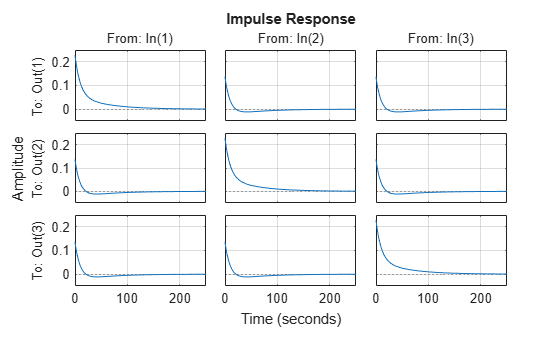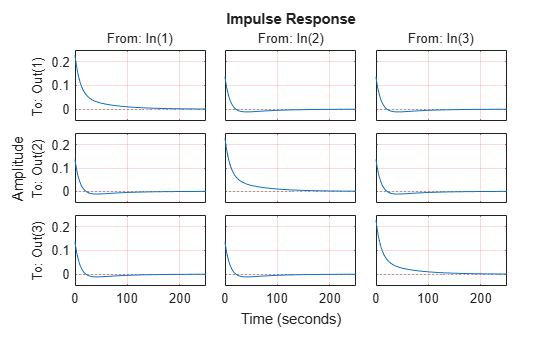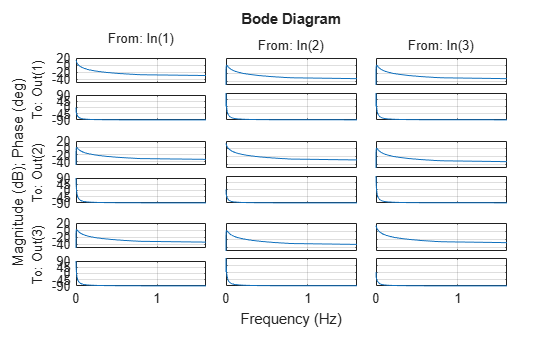setoptions

Set plot options handle or plot options property

Description

You can use setoptions to set the plot handle options or properties list and use it to customize the plot, such as modify the axes labels, limits and units. For a list of the properties and values available for each plot type, see Properties and Values Reference. To customize an existing plot using the plot handle:

1. Obtain the plot handle

2. Use getoptions to obtain the option set

3. Update the plot using setoptions to modify the required options

example

setoptions(h,p) sets preferences for response plot using the plot handle h is the plot handle and plot options handle p that contains information about plot options.

example

setoptions(h,'property1','value1',...,'propertyN','valueN') assigns values to property-value pairs instead of using the plot options handle p. For a list of the properties and values available for each plot type, see Properties and Values Reference.

setoptions(h,p,'property1','value1',...,'propertyN','valueN') first assigns properties using the plot options handle p, and then overrides any properties governed by the specified property-value pairs.. For a list of the properties and values available for each plot type, see Properties and Values Reference.

Examples

collapse all

For this example, consider a MIMO state-space model with 3 inputs, 3 outputs and 3 states. Create a impulse plot with red colored grid lines.

Create the MIMO state-space model sys_mimo.

J = [8 -3 -3; -3 8 -3; -3 -3 8];
F = 0.2*eye(3);
A = -J\F;
B = inv(J);
C = eye(3);
D = 0;
sys_mimo = ss(A,B,C,D);
size(sys_mimo)
State-space model with 3 outputs, 3 inputs, and 3 states.

Create an impulse plot with plot handle h and use getoptions for a list of the options available.

h = impulseplot(sys_mimo)h =

resppack.timeplot
p = getoptions(h)
p =

Normalize: 'off'
SettleTimeThreshold: 0.0200
RiseTimeLimits: [0.1000 0.9000]
TimeUnits: 'seconds'
ConfidenceRegionNumberSD: 1
IOGrouping: 'none'
InputLabels: [1x1 struct]
OutputLabels: [1x1 struct]
InputVisible: {3x1 cell}
OutputVisible: {3x1 cell}
Title: [1x1 struct]
XLabel: [1x1 struct]
YLabel: [1x1 struct]
TickLabel: [1x1 struct]
Grid: 'off'
GridColor: [0.1500 0.1500 0.1500]
XLim: {3x1 cell}
YLim: {3x1 cell}
XLimMode: {3x1 cell}
YLimMode: {3x1 cell}

Use setoptions to update the plot with the requires customization.

setoptions(h,'Grid','on','GridColor',[1 0 0]);The impulse plot automatically updates when you call setoptions. For MIMO models, impulseplot produces a grid of plots, each plot displaying the impulse response of one I/O pair.

For this example, consider a MIMO state-space model with 3 inputs, 3 outputs and 3 states. Create a Bode plot with linear frequency scale, specify frequency units in Hz and turn the grid on.

Create the MIMO state-space model sys_mimo.

J = [8 -3 -3; -3 8 -3; -3 -3 8];
F = 0.2*eye(3);
A = -J\F;
B = inv(J);
C = eye(3);
D = 0;
sys_mimo = ss(A,B,C,D);
size(sys_mimo)
State-space model with 3 outputs, 3 inputs, and 3 states.

Create a Bode plot with plot handle h and use getoptions for a list of the options available.

h = bodeplot(sys_mimo);
p = getoptions(h)
p =

FreqScale: 'log'
MagUnits: 'dB'
MagScale: 'linear'
MagVisible: 'on'
MagLowerLimMode: 'auto'
PhaseUnits: 'deg'
PhaseVisible: 'on'
PhaseWrapping: 'off'
PhaseMatching: 'off'
PhaseMatchingFreq: 0
ConfidenceRegionNumberSD: 1
MagLowerLim: 0
PhaseMatchingValue: 0
PhaseWrappingBranch: -180
IOGrouping: 'none'
InputLabels: [1x1 struct]
OutputLabels: [1x1 struct]
InputVisible: {3x1 cell}
OutputVisible: {3x1 cell}
Title: [1x1 struct]
XLabel: [1x1 struct]
YLabel: [1x1 struct]
TickLabel: [1x1 struct]
Grid: 'off'
GridColor: [0.1500 0.1500 0.1500]
XLim: {3x1 cell}
YLim: {6x1 cell}
XLimMode: {3x1 cell}
YLimMode: {6x1 cell}

Use setoptions to update the plot with the requires customization.

setoptions(h,'FreqScale','linear','FreqUnits','Hz','Grid','on');The Bode plot automatically updates when you call setoptions. For MIMO models, bodeplot produces an array of Bode plots, each plot displaying the frequency response of one I/O pair.

Create the following continuous-time transfer function:

$H\left(s\right)=\frac{1}{s+1}$

sys = tf(1,[1 1]);

Create a Bode plot with plot handle h.

h = bodeplot(sys);Create a plot options handle p.

p = getoptions(h);

Change frequency units of the plot to Hz.

p.FreqUnits = 'Hz';

Apply the plot options to the Bode plot.

setoptions(h,p);Alternatively, use setoptions(h,'FrequencyUnits','Hz').

Input Arguments

collapse all

Plot handle, specified as a plot handle object. For example, h is a mpzplot object for a pole-zero or I/O pole-zero plot.

Plot options handle, specified as a plot options handle object. For example, p is a PZMapOptions object for a pole-zero or I/O pole-zero plot.

There are two ways to create a plot options handle:

• Use getoptions, which accepts a plot handle and returns a plot options handle.

p = getoptions(h)

• Create a default plot options handle using one of the following commands:

For example,

p = bodeoptions

returns a plot options handle for Bode plot.